## Tamilnadu Samacheer Kalvi 10th Maths Solutions Chapter 1 Relations and Functions Additional Questions

Question 1.
Let A = {1, 2, 3, 4} and B = {-1, 2, 3, 4, 5, 6, 7, 8, 9, 10, 11, 12} Let R = {(1, 3), (2, 6), (3,10), (4, 9)} ⊂ A × B be a relation. Show that R is a function and find its domain, co-domain and the range of R.
Domain of R = {1, 2, 3, 4}
Co-domain of R = B = {-1, 2, 3, 4, 5, 6, 7, 9, 10, 11, 12}
Range of R= {3, 6, 10, 9}

Question 2.
Let A = {0, 1, 2, 3} and B = {1, 3, 5, 7, 9} be two sets. Let f: A → B be a function given by f(x) = 2x + 1. Represent this function as (i) a set of ordered pairs (ii) a table (iii) an arrow and (iv) a graph.
Solution:
A = {0, 1, 2, 3}, B = {1, 3, 5, 7, 9}
f(x) = 2x + 1
f(0) = 2(0) + 1 = 1
f(1) = 2(1) + 1 = 3
f(2) = 2(2) + 1 = 5
f(3) = 2(3) + 1 = 7
(i) A set of ordered pairs.
f = {(0, 1), (1, 3), (2, 5), (3, 7)}
(ii) A table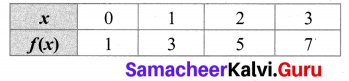(iii) An arrow diagram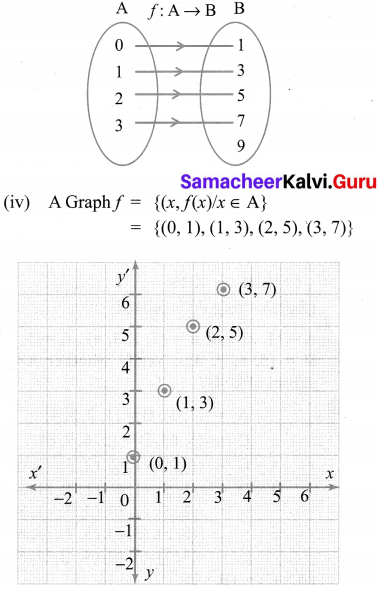Question 3.
State whether the graph represent a function. Use vertical line test.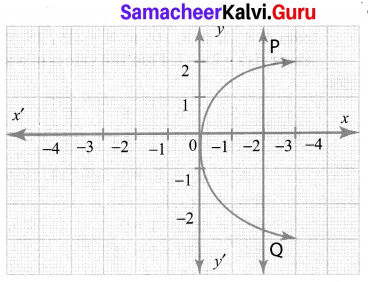Solution:
It is not a function as the vertical line PQ cuts the graph at two points.

Question 4.
Let f = {(2, 7), (3, 4), (7, 9), (-1, 6), (0, 2), (5, 3)} be a function from A = {-1, 0, 2, 3, 5, 7} to B = {2, 3, 4, 6, 7, 9}. Is this (i) an one-one function (ii) an onto function, (iii) both one- one and onto function?
Solution:
It is both one-one and onto function.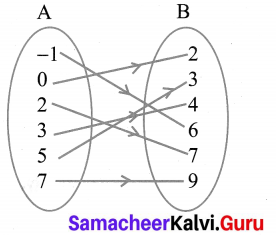All the elements in A have their separate images in B. All the elements in B have their preimage in A. Therefore it is one-one and onto function.

Question 5.
A function f: (-7,6) → R is defined as follows.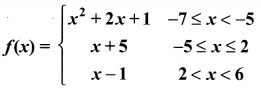Find (i) 2f(-4) + 3 f(2)
(ii) f(-7) – f(-3)
Solution: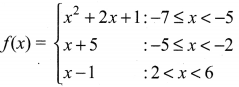(i) 2f(-4) + 3f(2)
f(-4) = x + 5 = -4 + 5 = 1
2f(-4) = 2 × 1 = 2
f(2) = x + 5 = 2 + 5 = 7
3f(2) = 3(7) = 21
∴ 2f(-4) + 3f(2) = 2 + 21 = 23

(ii) f(-7) = x2 + 2x + 1
= (-7)2 + 2(-7) + 1
= 49 – 14 + 1 = 36
f(3) = x + 5 = -3 + 5 = 2
f(-7) – f(-3) = 36 – 2 = 34Question 6.
If A = {2,3, 5} and B = {1, 4} then find
(i) A × B
(ii) B × A
A = {2, 3, 5}
B = {1, 4}

(i) A × B = {2,3,5} × {1,4}
= {(2, 1) (2, 4) (3, 1) (3, 4) (5,1) (5, 4)}.

(ii) B × A = {1,4} × {2,3,5}
= {(1,2) (1,3) (1,5) (4, 2) (4, 3) (4, 5)}

Question 7.
Let A = {5, 6, 7, 8};
B = {- 11, 4, 7, -10, -7, – 9, -13} and
f = {(x,y): y = 3 – 2x, x ∈ A, y ∈ B}.
(i) Write down the elements of f.
(ii) What is the co-domain?
(iii) What is the range?
(iv) Identify the type of function.
Given, A = {5, 6, 7, 8},
B = {- 11,4, 7,-10,-7,-9,-13}
y = 3 – 2x
ie; f(x) = 3 – 2x
f(5) = 3 – 2 (5) = 3 – 10 = – 7
f(6) = 3 – 2 (6) = 3 – 12 = – 9
f(7) = 3 – 2(7) = 3 – 14 = – 11
f(8) = 3 – 2 (8) = 3 – 16 = – 13
(i) f = {(5, – 7), (6, – 9), (7, – 11), (8, – 13)}
(ii) Co-domain (B)
= {-11,4, 7,-10,-7,-9,-13} i
(iii) Range = {-7, – 9, -11,-13}
(iv) It is one-one function.

Question 8.
A function f: [1, 6] → R is defined as follows: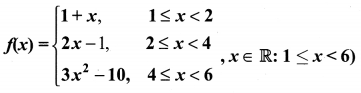Find the value of (i) f(5)
(ii) f(3)
(iii) f(2) – f(4).
Solution: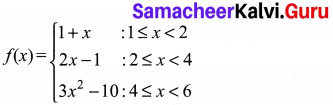(i) f(5) = 3x2 – 10
= 3 (52) – 10 = 75 – 10 = 65
(ii) f(3) = 2x – 1
= 2(3) – 1 = 6 – 1 = 5
(ii) f(2) – f(4)
f(2) = 2x – 1
= 2(2) – 1 = 3
f(4) = 3x2 – 10
= 3(42) – 10 = 38
∴ f(2) – f(4) = 3 – 38 = 35Question 9.
The following table represents a function from A = {5, 6, 8, 10} to B = {19, 15, 9, 11}, where f(x) = 2x – 1. Find the values of a and b.
Solution: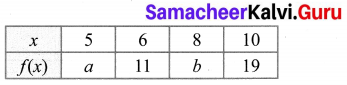A = {5, 6, 8, 10}, B = {19, 15, 9, 11}
f(x) = 2x – 1
f(5) = 2(5) – 1 = 9
f(8) = 2(8) – 1 = 15
∴ a = 9, b = 15

Question 10.
If R = {(a, -2), (-5, 6), (8, c), (d, -1)} represents the identity function, find the values of a,b,c and d.
Solution:
R = {(a, -2), (-5, b), (8, c), (d, -1)} represents the identity function.
a = -2, b = -5, c = 8, d = -1.